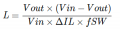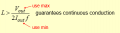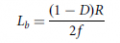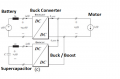# How do i select inductor for DC-DC converter (buck converter)

#### BramLabs

Joined Nov 21, 2013
98
Oh yeah sir, let's just say, i have specification like this :
Fsw = 40 KHz
Vin = 12 V
Vout = 6 V
Rout = 5 ohm
Output Ripple (delta Vout) = 1%

First :Second :Third :How to choose between three of them sir, which one worked best for controlling electrical motor (EV application or HEV).

Thank you before !

Last edited:

#### MrAl

Joined Jun 17, 2014
10,587
Hello,

The relationship would be based on:
V=L*di/dt

and solving for L:
L=V*dt/di

V is based on the input/output relationship for a constant output voltage:
V=Vin-Vout

dt would the the charge time or fall time, whichever is longer.
di is the change from min to max current, which normally looks like a triangle wave.

There is a catch though, and that is the inductor must be able to handle the required average DC current without saturating. Going higher on the rating is better to prevent problems with saturation. Looking up the spec's on the inductor you should be able to find this.

Just one question, you say you have 0.5 amp but if the output is 6v and load 5 ohms then the average DC output current is over 1 amp. What did you mean by 0.5 amps?

•BramLabs

#### BramLabs

Joined Nov 21, 2013
98
Hello,

The relationship would be based on:
V=L*di/dt

and solving for L:
L=V*dt/di

V is based on the input/output relationship for a constant output voltage:
V=Vin-Vout

dt would the the charge time or fall time, whichever is longer.
di is the change from min to max current, which normally looks like a triangle wave.

There is a catch though, and that is the inductor must be able to handle the required average DC current without saturating. Going higher on the rating is better to prevent problems with saturation. Looking up the spec's on the inductor you should be able to find this.

Just one question, you say you have 0.5 amp but if the output is 6v and load 5 ohms then the average DC output current is over 1 amp. What did you mean by 0.5 amps?
Hello again MrAl ^^
Yeah, i know the relationship of V=L*di/dt
Let me just derive your relationship (because i prefer to use the third equation in my question) :
L = V *dt/di
Because V = Vin - Vout
L = (Vin - Vout)*dt/di
and for charging mode, the integration of dt would be from 0 to T, so dt = T
L = (Vin - Vout)*T/di
And because we can say Vout = D*Vin ; D=duty cycle
And also di = ripple of the current (which in your case, you say the current 'looked' like a triangle wave)
L = Vin*(1 - D)*T/di
T = 1/f, so :
L = Vin*(1-D)/di*f
Because Vin / di can be R, so :
L = R(1-D)/f
It looks like almost same as the third question, but i missed the "2" part. ahahaha... sorry sir.
maybe you can help me how to get the "2" part.

Oh yeah, btw, i found the third equation in M.H. Rashid's Book
And the other 2 at google. So i prefer to choose the equation based on book, not the google. ahahha..

I want to control the current of the electrical motor like the picture below :So, let's just say, i want to limit my battery current for 1 Amp, and if the motor NEEDS more current (by applying acceleration), the motor will normally sink the current more from the battery, let's just say 0.5 Amp. And i want to control the current through the motor, so that the battery will only supply 1 Amp and the remaining current NEEDED will be the supercapacitor's job.
And and the 'controller' i write a code :
1). Capacitor should be fully charged
2). If the current at the motor UNDER or BELOW 1 Amp, DC-DC Buck/Boost converter (at the bottom) not active
3). If the current at the motor over 1 Amp, CHECK if the Voltage at supercapacitor between the range of Minimum Voltage Supercapacitor to Maximum Voltage Supercapacitor (Let's say minimum = 4 Volt and Maximum = 9 Volt). If yes, then Boost converter active (so the current will flow from supercapacitor to motor), If no, buck converter active (the battery charge the supercapacitor).

Yeah, the average DC output current would be 1 Amp to charge the super capacitor. (buck converter mode)

Big thanks for the information sir.

#### MrAl

Joined Jun 17, 2014
10,587
Hello again,

Oh you are welcome, i help whenever i can.

The extra '2' comes from converting from the full current excursion to the peak current excursion. So if the full change in current was 0.5 amps, then the peak to peak ripple current is 0.5 amps but the peak current is only half of that, which is 0.25 amps. They chose to calculate the peak current by including that '2' in the denominator.

To make this more clear we could do a full analysis of the circuit, but because of the initial voltage and current the equation for the inductor current comes out a little longer than we like to see sometimes, so i'll post here the solution that we get using a 50 percent duty cycle:
IL(t)=(e^(-t/(2*C*R))*(((2*C*L*((C*E*R^2)/2-E*L)+C*E*L^2)*sin((t*sqrt(L*(4*C*R^2-L)))/(2*C*L*R)))/sqrt(L*(4*C*R^2-L))-C*E*L*cos((t*sqrt(L*(4*C*R^2-L)))/(2*C*L*R))))/(C*L*R)
+E/R

This is the inductor current over time with a 50 percent duty cycle wave of amplitude E volts.
The other variables are:
C is the output filter cap,
L is the inductor value,
t is the 'on' time, which BTW makes the inductor current make a full excursion from min to max.

To appreciate an equation like that you probably have to have some software that can handle that, and set the time equal to 1/2 of the switching cycle time.
The expression that handles the initial current and voltage is longer and to appreciate that you'd need software to handle it too, so i'll wait to post that one if you care to look into this.

Also noteworthy is my electrical engineering handbook quotes the formula with the '2' in the denominator too, and specifically states that it is the peak current not the peak to peak current.

For a numerical example, with E=10v (a 10v square wave on for 50 percent of the time) and L=50uH and C=10uf and R=10 ohms and switching cycle time of 10us (which makes the 'on' time 5us), the inductor current excursions go plus and minus 0.25 amps, so one full excursion is 0.5 amps. That means the peak to peak ripple is 0.5 amps.

#### BramLabs

Joined Nov 21, 2013
98
Hello again,

Oh you are welcome, i help whenever i can.

The extra '2' comes from converting from the full current excursion to the peak current excursion. So if the full change in current was 0.5 amps, then the peak to peak ripple current is 0.5 amps but the peak current is only half of that, which is 0.25 amps. They chose to calculate the peak current by including that '2' in the denominator.

To make this more clear we could do a full analysis of the circuit, but because of the initial voltage and current the equation for the inductor current comes out a little longer than we like to see sometimes, so i'll post here the solution that we get using a 50 percent duty cycle:
IL(t)=(e^(-t/(2*C*R))*(((2*C*L*((C*E*R^2)/2-E*L)+C*E*L^2)*sin((t*sqrt(L*(4*C*R^2-L)))/(2*C*L*R)))/sqrt(L*(4*C*R^2-L))-C*E*L*cos((t*sqrt(L*(4*C*R^2-L)))/(2*C*L*R))))/(C*L*R)
+E/R

This is the inductor current over time with a 50 percent duty cycle wave of amplitude E volts.
The other variables are:
C is the output filter cap,
L is the inductor value,
t is the 'on' time, which BTW makes the inductor current make a full excursion from min to max.

To appreciate an equation like that you probably have to have some software that can handle that, and set the time equal to 1/2 of the switching cycle time.
The expression that handles the initial current and voltage is longer and to appreciate that you'd need software to handle it too, so i'll wait to post that one if you care to look into this.

Also noteworthy is my electrical engineering handbook quotes the formula with the '2' in the denominator too, and specifically states that it is the peak current not the peak to peak current.

For a numerical example, with E=10v (a 10v square wave on for 50 percent of the time) and L=50uH and C=10uf and R=10 ohms and switching cycle time of 10us (which makes the 'on' time 5us), the inductor current excursions go plus and minus 0.25 amps, so one full excursion is 0.5 amps. That means the peak to peak ripple is 0.5 amps.
Wow.....wow....wow..... wait...wait.... sir.
I haven't ready for that equation. ahahahaha.
It seems look harder than i thought.
But maybe, if i write it in a good term, i can see the pattern (looking the equation through my monitor, would turn my eyes into tears. ahahahaha)

Oh yeah sir, i want to ask againMay i ? (you should answer me)

Let's just say in my case :
- The current at the motor over 1 Amp, and the microcontroller check the minimum voltage of the supercapacitor and maximum voltage of the super capacitor. If it's IN THE RANGE (in my case between 4 V - 9 V), then the boost converter active.

What i want to ask is that, for the boost converter, R out is about 2 ohm (in this boost converter mode, R out is the motor itself).
And the AVERAGE CURRENT, would be I average = V out / Rout = 12 / 2 = 6 Amp (in my case, the Vout is 12 ; Vout = V motor)
The question is, i just need 0.5 Amp. And the average current would be 6 Amps in this case. I can 'set' the 'Amp' by changing the duty cycle isn't it ?
So i mean, by applying various duty cycle (let's just say, from 20% to 80%), i could see, the best way to 'set' the current by 'looking at the truth table' isn't it ?
From the data, i knew by applying duty cycle, let's just say 40%, gives 0.5 Amps (if the motor needed 0.5 Amp), and if the motor needed more than 0.5 Amps, by changing the duty cycle, will give more current isn't it ?

Thank you sir for your time ^^

#### MrAl

Joined Jun 17, 2014
10,587
Hi,

You're welcome, and no problem. When i help people with their circuits i get to see them learn more and i learn more too because since i dont work in the industry anymore i dont use this stuff every day anymore and so i tend to forget some things, and by helping out a little i refresh what i have covered in the past and thus remember the interesting things about these circuitsIf i understand you right, you want to regulate the current out to the motor by measuring the current and then adjusting the drive in discrete steps?
So for example if the current is 0.5 amps and you want more current, you would step it up to maybe 0.6 amps, then 0.7 amps. etc.

The equations for these kinds of circuits end up being in a form like:
e(-a*t)*(A*sin(wt)+B*cos(wt))

which you may or may not want to convert into e(-a*t)*C*sin(wt+ph).

So it's just a damped sinusoidal.

The tricky part is that the drive signal is a square wave so the initial conditions also have to be solved for and included in the transfer function. This creates a more complex equation than if we didnt need the initial conditions.
But i did not mean to imply that you NEED to use this type of analysis if you are satisfied with using the equation with the '2' in the denominator. That gives you the inductance for a 50 percent duty cycle and that usually is good enough. It's only when you want to look closer that you have to do a little more analysis, but what you end up with is better than you would see in a simulator using all ideal components. So this problem partly depends on how satisfied you are with the equation you already have and how badly you want to know more about it•BramLabs

#### MrAl

Joined Jun 17, 2014
10,587
Hi,

I just realized that two of the formulas have a '2' in the bottom, so let me write out the formula now...

L=Vs*(1-D)/(2*di*f)

Here, Vs is the input source voltage,
D is the duty cycle expressed as a fraction (so 50 percent is 0.5, 10 percent is 0.10),
di is the peak to peak ripple current,
f is the switching frequency.

Since a 50 percent duty cycle is often used, this gives us:
L=Vs/(4*di*f)

So with Vs=10 and di=1/2 and f=100kHz, we get L=50uH.

Also of interest is the capacitor ripple voltage, which comes from knowing the ripple current:
dv=i*dt/C

dv=di/(8*f*C)

where di is the ripple current, f is the switching frequency, and C the output capacitance.
So with a peak to peak ripple current of 1/2, f=100kHz, C=12.5uf, the ripply voltage peak to peak is 50mv.
This is just an estimate and in reality it might be a little lower than that, but i'll have to do the more accurate calculation a little later today.

LATER:
Ok verified the formula for dv and here is the other form:
L*C=(Vs*(1-D)*D)/(8*dv*f^2)

C=(Vs*(1-D)*D)/(8*L*dv*f^2)

where
Vs is the input source voltage, D the duty cycle as a fraction (like 0.5 for 50 percent), dv is the desired peak to peak ripple voltage, f is the frequency in Hertz.

So for a numerical example, if we have D=0.5 again and we want a peak to peak ripple voltage of 50mv then dv=0.050, and with L=50uH (from the previous calculation using di) and f=100kHz, we get C=12.5uf.

So between the two formulas we managed to calculate the required L to meet the desired ripple current, and then calculated C to meet the desired ripple voltage.

A little shortcut given a 50 percent duty cycle would be:
C=Vs/(32*L*dv*f^2)

As a side note, the estimation for ripple voltage comes from using a square wave current, but the more realistic wave is a triangle shaped wave. Interestingly, the peak to peak voltage comes out the same either way it's just that one waveshape is triangular and one is a piece wise t^2 shaped wave that closely resembles a sinusoidal wave.

Last edited:
•BramLabs